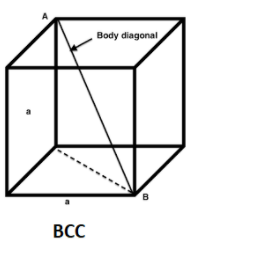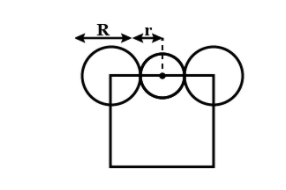# The radius of the largest sphere which fits properly at the center of the edge of body centered cubic unit cell is: (edge length is represented by a)(A) 0.134a(B) 0.027a(C) 0.067a(D) 0.047aVerified
176.4k+ views
Hint: A unit cell is the smallest repeating unit in the crystal lattice, it is the building block of a crystal like the cell which is the building block of the human body. Body centered cubic cell is one of the types of the unit cell.

Complete step by step solution:
To solve this question we have to find the relation between the radius (r) and the edge of the body centered unit cell (a). We know that the total number of atoms present in the body centered cubic cell is = 2.In body centered unit cells, atoms in the body diagonals are in contact with each other. So the length of the body diagonal is = $R+2R+R=4R$
The body diagonal = $\sqrt{3}a$
So, 4R = $\sqrt{3}a$---1
According to the question$\dfrac{a}{2}=\left( R+r \right)$---2
From equation 1 and 2
$\dfrac{a}{2}=\dfrac{a\sqrt{3}}{4}+r$
$a(\dfrac{2-\sqrt{3}}{4})=r$
r = 0.067a

Hence the correct answer is option (C)

Note: In the body centered unit cell, atoms are present at the center and at the corner of the cube. And as we know that there is only one center in a cube hence only one atom is completely present there that means that this atom is not shared with any other unit cell and one atom at the corner contributes to eight other corners. So the total number of atoms present in the body centered cubic cell is = 2. i.e.
1 atom at body center $+\text{ (}\dfrac{1}{8})(8)$ at the corner = 2 atoms